# Symmetry on a set

(diff) ← Older revision | Latest revision (diff) | Newer revision → (diff)A non-negative real-valued functiondefined on the set of all pairs of elements ofand satisfying the following axioms:

1)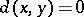if and only if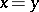;

2)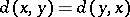for any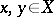.

In contrast to a metric and a pseudo-metric, a symmetry need not satisfy the triangle axiom. Relative to a symmetryon a setthere is a topology defined on: A set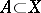is closed (relative to) if and only iffor each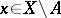. Here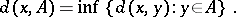The closure of a setin this topological space contains the set of allfor which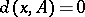but need not be exhausted by this set. Correspondingly, the-ball around a point ofmay have an empty interior. A topological space is called symmetrizable if its topology is generated by the above rule from some symmetry. The class of symmetrizable spaces is much wider than the class of metrizable spaces (cf. Metrizable space): A symmetrizable space need not be paracompact, normal or Hausdorff. In addition, a symmetrizable space need not satisfy the first axiom of countability.

However, each symmetrizable space is sequential, that is, its topology is determined by convergent sequences by the rule: A setis closed if and only if the limit of each sequence of points ofthat converges inbelongs to. For compact Hausdorff spaces symmetrizability is equivalent to metrizability.

How to Cite This Entry:
Symmetry on a set. Encyclopedia of Mathematics. URL: http://encyclopediaofmath.org/index.php?title=Symmetry_on_a_set&oldid=14963
This article was adapted from an original article by A.V. Arkhangel'skii (originator), which appeared in Encyclopedia of Mathematics - ISBN 1402006098. See original article